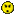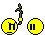# Cata char skins / details etc

Status
Not open for further replies.

#### boppas

##### Fledgling Freddie
ellos peepsAnyone have any links for good detailed descriptions & pics on the new skins from catacombs for our current chars, the official euro webby says little (as usual) & is fkin useless tbh

Also im guessing that we will be able to choose color/hieght etc for our current chars as there will be big changes to what we already have & we might not like the way we look?

One last thing..

99% of my chars are small.. Luri's/Kobbie's will these remain small or do they get....stretched! ? ...any links to pics much appreciated, tyBops#### Chimaira

##### Can't get enough of FH
Kobolds are taller.

much taller tbh

Lurikeens seems small as before

Also you can edit most on the face for your character. its still small medium large that counts on size

You can alter the nose etc by strechin this bar. same with chin eyes etc

#### vintervargen

##### Banned
and they've made the NF boats go slower so we can enjoy the view better =)))))))))))))))))))))))))))))))))))))))))))))))))))))))))))))))))))))))))))))))))))))))))))))))))))))))))))))))))))))))))))))))))))))))))))))))))))))))))))))))))))))))))))))))))))))))))))))))))))))))))))))))))))))))))))))))))))))))))

and removed all hasteners, after all its a roleplaying game! =))))))))))))))))))))))))))))))))))))))))))))))))))))))))))))))))))))))))))))))))))))))))))))))))))))))))))))))))))))))))))))))))))))))))))

and also you cannot port between keeps anymore, because that would save you time =))))))))))))))))))))))))))))))))))))))))))))))))))))))))))))))))))))))))))))))))))))))))))))))

and not only can you get rr12, but also ML20! more fantastic thrilling adventures lies ahead! and we promise these abilities wont affect RvR in any way! =))))))))))))))))))))))))))))))))))))))))))))))))))))))))))))))))))))))))))))))))))))))))))))))))))))))))))))))))))))))))))))))))))))))))))))))))))))))))))))))))))))))))))))

#### boppas

##### Fledgling Freddie
Kobbies are much tallerwtf they change that for..

So, do we get a choice of another small char? , Hibs its Luri / Albs its inco so wtf do mids get ?

Why do they have to change the sizes etc, Most of us that are left playing DAoC created our chars many moons ago... tbh i cant stand playing tall chars!

As ive said, most of my chars are small & i much prefer them. I spend time choosing the race/looks etc as i guess most of us do when we make a new char, afterall we are stuck wit it for good... (until some thick c00nt at mythic decides to change the size of a race 100%), Can you imagine what people would think if they decided to make a Celt/Norse & Armsman luri sized! ..fk sake, I made kobbies purely because they were smallwell played mythic... another f00k up!

Yup im QQ'nIf i wanted a tall char, i would have rolled one. Why dont they change/fix things that are USEFUL for a change.... Roll on DnL.

DAoC - Dark Age Of ChangeAlot ....muppets#### cemi0

##### Can't get enough of FH
Lol, would you look at that whine?
Why dont you take a breath, a chill pill and wait for Catacombs to see what they changed themself instead of instantly believing what some random guy (no offend) says?

Random Guy!

#### Huntingtons

##### Resident Freddy
Camelot Herald said:
The Vampiir class is a fighter who wields a one-handed piercing weapon in its right hand. While in melee combat, it is able to cast Vampiir magic with the left hand. The class does not have a normal power pool, however. It will gain power from a variety of attacks - primarily melee strikes. The class receives Safefall and Climb Walls, as well as a passive form of health regeneration. Vampiiric magic comes in three categories or spell lines: "Self Enhancement" spells such as parry, evade, run speed, magic and melee absorption bonuses; "Offensive Damage" spells such as Lifetaps, Flame Strikes, and a special ability to turn the left hand into a claw for further melee attacks; and "Utility" spells such as a single target mez, snare, Lull Enemies, and effectiveness debuffs.

i like how they couldn't make a background story up for the vampir and banshee, what a load of bull tbh... fucking noobs at mythic...

#### Dubaxter

##### Fledgling Freddie
Just stop whining you bunch of morons. If you dont like the game piss off to wow.

#### Gamah

##### Banned
Whos whining? I dont see anyone having a moan...

#### Gamah

##### Banned
Huntingtons said:
i like how they couldn't make a background story up for the vampir and banshee, what a load of bull tbh... fucking noobs at mythic...
its obvious they come from the underground !!!!!!!!!!!!!!!!!!!!!!!!!!!!!!!!!!!!!!!!!!!!!!!!!!!!!!!!!!!!!!! tis; like the whole point of the expantion !#### Shanaia

##### Fledgling Freddie
I'm telling you every day these boards start looking more and more like VN boards...#### Litmus

##### FH is my second home
TALL KOBBIES?!?!, Mid Nerf"""!!!

#### Flimgoblin

##### It's my birthday today!
http://camelot-europe.goa.com/en/

click on "expansions" then "catacombs" then "graphics" voila... info about the catacombs expansion graphics, heaven forbid.

oh and kobolds are all now 9 feet tall, as are lurikeen and inconnu, to offset this half ogres, trolls and firbolg have all been shrunk to 3' tall.

#### Flimgoblin

##### It's my birthday today!
feel free to repost your request for information with less of the whine.

Status
Not open for further replies.